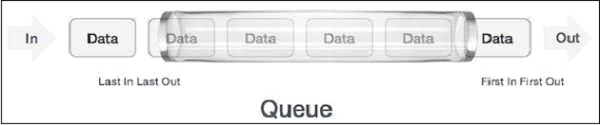# Queue Data Structure in Javascript

In this article, we are going to discuss the queue data structure in JavaScript. It is a linear data structure where the enqueue and dequeue of elements follow the FIFO (first in first out sequence). The queue is open at both ends. One end is always used to insert data and the other is used to remove data. Here, we use two pointers rear and front. The rear is used to insert the data and the front is used to remove the data.

A real-world example of the queue can be a single-lane one-way road, where the vehicle enters first, exits first.The following diagram shows how a queue works −## Example 1

The following example demonstrates how to implement a queue class data structure in JavaScript. We are also going to show the use of enqueue(), peek(), and size() methods of the queue.

<!DOCTYPE html>
<html lang="en">
<meta charset="UTF-8" />
<meta http-equiv="X-UA-Compatible" content="IE=edge" />
<meta name="viewport" content="width=device-width, initial-scale=1.0" />
<title>Queue Data Structure</title>
<body>
<script type="text/javascript">
class Queue {
constructor() {
this.queArr = [];
this.rearIdx = 0; //tail
}
enqueue(elem) {
this.queArr[this.rearIdx] = elem;
this.rearIdx++;
}
dequeue() {
if (this.isEmpty()) {
document.write("Queue Underflow..!</br>");
return;
}
let elem = this.queArr[this.frontIdx];
delete this.queArr[this.frontIdx];
this.frontIdx++;
return elem;
}
peek() {
return (
"The peek element of the Queue is : " +
this.queArr[this.frontIdx] +
"</br>"
);
}
size() {
document.write("The size of the Queue is : ");
return this.rearIdx - this.frontIdx;
}
isEmpty() {
document.write("<br>");
return this.queArr.length == 0;
}
display() {
if (this.queArr.length !== 0) {
return "The Queue elements are : " + this.queArr + "</br>";
} else {
document.write("The Queue is Empty..! <br>");
}
}
clear() {
document.write("</br>The Queue is cleared..!" + "<br>");
this.queArr = [];
}
}
let queue = new Queue();
queue.enqueue(1);
queue.enqueue(2);
queue.enqueue(3);
queue.enqueue(4);
queue.enqueue(5);
document.write(queue.display());
document.write(queue.peek());
document.write(queue.size());
</script>
</body>
</html>


## Example 2

The following example demonstrates how to implement a queue class data structure in JavaScript. And also discuss how to perform various operations on the queue elements in order to either modify or check the status of the queue.

<!DOCTYPE html>
<html lang="en">
<meta charset="UTF-8" />
<meta http-equiv="X-UA-Compatible" content="IE=edge" />
<meta name="viewport" content="width=device-width, initial-scale=1.0" />
<title>Queue Data Structure</title>
<body>
<script type="text/javascript">
class Queue {
constructor() {
this.queArr = [];
this.rearIdx = 0; //tail
}
enqueue(elem) {
this.queArr[this.rearIdx] = elem;
this.rearIdx++;
}
dequeue() {
if (this.isEmpty()) {
document.write("Queue Underflow..!</br>");
return;
}
let elem = this.queArr[this.frontIdx];
delete this.queArr[this.frontIdx];
this.frontIdx++;
return elem;
}
peek() {
return (
"The peek element of the Queue is : " +
this.queArr[this.frontIdx] +
"</br>"
);
}
size() {
document.write("The size of the Queue is : ");
return this.rearIdx - this.frontIdx;
}
isEmpty() {
document.write("<br>");
return this.queArr.length == 0;
}
display() {
if (this.queArr.length !== 0) {
return "The Queue elements are : " + this.queArr + "</br>";
} else {
document.write("The Queue is Empty..! <br>");
}
}
clear() {
document.write("</br>The Queue is cleared..!" + "<br>");
this.queArr = [];
}
}
let queue = new Queue();
queue.enqueue(1);
queue.enqueue(2);
queue.enqueue(3);
queue.enqueue(4);
queue.enqueue(5);
document.write(queue.display());
document.write(queue.peek());
document.write(queue.size());
queue.clear();
queue.display();
</script>
</body>
</html>